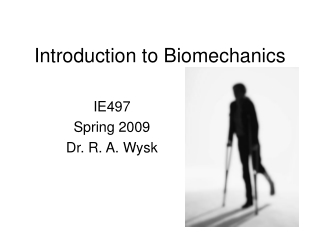DownloadDownload PresentationIntroduction to Biomechanics

# Introduction to Biomechanics

Télécharger la présentation## Introduction to Biomechanics

- - - - - - - - - - - - - - - - - - - - - - - - - - - E N D - - - - - - - - - - - - - - - - - - - - - - - - - - -
##### Presentation Transcript

1. Introduction to Biomechanics IE497 Spring 2009 Dr. R. A. Wysk

2. What is Biomechanics? • The process of describing, analyzing and assessing human motion • Some focus on handicapped • Gate of motion • Joints and joint replacement • Stable and sustainable

3. Examples

4. Flow of joint position from a camera

5. The Arm

6. Joint Replacement Design

7. An Elbow Joint

8. Use a free-body diagram

9. Free-body diagrams • Shows all forces and kinematic points • Draw a representative free-body diagram • Equilibrium mapping • Write the equations of static equilibrium • Solve/analyze the system

10. Our diagram Theequations for static equilibrium are: SM = 0 SFx = 0 SFy = 0

11. Our problem

12. Hip Biomechanics